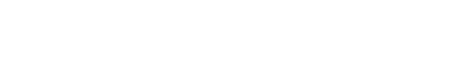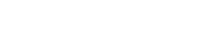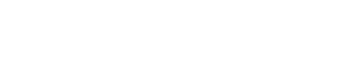## CHEMISTRY PAPER 1 - 2019 KCSE KASSU JOINT MOCK EXAMS (QUESTIONS AND ANSWERS)

Instructions to candidates

• Answer all questions in this question paper.
• Answers to all questions must be written in the spaces provided in this booklet.
• This paper consists of Two Sections A and B in the spaces provided.
• All working MUST be clearly shown.
• Electronic calculators and mathematical tables may be used.
• KNEC mathematical tables and non-programmable silent electronic calculators may be
1. State three differences between physical and chemical changes (3 Mark)
 Physical changes Chemical changes
1. Use the information  in the table below to answer the question that follow.(the letters do not represent the actual symbols  of the elements
 Element B C D E F Atomic number 18 5 3 5 20 Mass number 40 10 7 11 40

Which two letters represent the same element? Give a reason (1 Mark)
The table below shows the mass numbers and percentage abundances of isotopes W1 and W2 of element W
 Isotope Mass numbers % Abundance W1 12 98.3 W2 14 1.7

Determine the relative atomic mass of element W (2 Marks)
1. When carbon (IV) oxide gas was passed through aqueous calcium hydroxide a white suspension was formed.
1. Write an equation for the reaction that took place (1 Mark).
2. State and explain the observations that would occur when excess carbon (IV) oxide is bubbled through the white suspension (2 Marks)
1. The reaction below takes place on the haber process in the production of ammonia
N2(g) + 3H2 (g)  → 2 NH3 (g)
1. How the yield of ammonia would be affected if the pressure is increased. Give a reason (2 marks)
2. The forward reaction was found to be favored by decrease in temperature. What is the sign of  ∆H  on the above reaction (1 mark)
1. Describe how you would separate a mixture of iron fillings, iron (iii) chloride and sodium chloride (3 marks)
1. The pH values of solutions K,L,M,N and Pare as shown below
 Solution K L M N P pH  value 5 2 7 12 9

1. Which of the solution is likely to be
1. Soap solution                                      ( ½ mk)
2. Sodium chloride                                  ( ½ mk)
2. Zinc oxide was found to react with both solutions L and N. What property of zinc oxide was displayed y these reactions (1 mark)
1.
1. State Boyles law of gases (1 mark)
2. In a class experiment, it was found that a gas occupied 48dm3 at 250 C and 2 atmospheres pressure. What volume would it occupy at STP (2 marks)
1. The set up below was used to prepare oxygen gas. Study it and answer the questions that follow1. Complete the diagram to show how oxygen gas is collected (2 marks)
2. Identify X ( mark)
3. Name two other reagents which can be reacted to produce oxygen gas in the laboratory ( mark)
1. Name the process which takes place when
1. Iodine changes directly from solid to gas (1 mark)
2. Fe2+( aq) changes to Fe3+(aq) (1 mark)
3. White sugar changes to black when mixed with concentrated sulphuric (VI) acid (1 mark)
1. 12.0cm3 of 0.05 M hydrochloric acid reacted with calcium hydrogen carbonate to form calcium chloride, water and carbon (IV) oxide.
1. Write the chemical equation for the reaction (1 mark)
2. Calculate the number of moles of hydrochloric acid used (1 mark)
3. Determine the number of moles of calcium hydrogen carbonate used (1 mark)
1. In the last stage of the solvay process, a mixture of sodium hydrogen carbonate and ammonium chloride is formed
1. State the method of separation used (1 mark)
2. Write an equation showing how lime is slaked (1 mark)
3. Name the by- product recycled in the above process ( 1 mark)
1. An akkanol has the following composition by mass: Hydrogen 13.5%, Oxygen 21.6% and carbon 64.9%
1. Determine the empirical formula of the alkanol (C = 12.0, H= 1.0, 0= 16.0 ) (2 marks)
2. Given that the empirical formula and molecular formula are the same, draw the open structure of the alkanol (1 mark)
1. The graph below shows how electrical conductivity of metals varies with temperature1. Explain the variation of electrical conductivity with temperature (2 marks)
2. State the particle responsible for conductivity in
1. Molten lead (ii) bromide      ( ½ mk)
2. Graphite         ( ½ mk)
1. Study the scheme and answer the questionsIdentify ;
1. Solid Z     (1 mk)
2. Substance P    (1 mk)
3. Give one use of substance P (1 mark)
1. The diagram below is a section of a model of the structure of element K1. State the type of bonding that exist in K
2. In which group of the periodic table does element K belong.  Give a reason (2 marks)
1. Study the diagram below and answer the questions that follow1. State two observations made in the above experiment when sodium react with water (2 marks)
2. Write a chemical equation for the reaction that takes place (1 mark)
1. Study the nuclear reactions below1. Identify emissions X and Y          (1 mk)
X
Y
2. 100g of radioactive substance reduced to 6.25g in 8 years. Calculate the half life of radioactive substance (2 marks)
1. Describe how a solid sample of lead (ii) chloride can be prepared using the following reagents: dilute nitric (v) acid, dilute hydrochloric acid, and lead (ii) carbonate powder (3 marks)
1. Explain why permanent hardness in water cannot be removed by boiling (2 marks)
2. Name two methods that can be used to remove permanent hardness from water (1 mark)
1. The diagram below shows the Frasch process used for extraction of sulphur. Use it to answer the questions that follow1. Identify X                                                                               (1 mark)
2. Why is it necessary to use super heated water in this process (1 Mark)
3. State one physical property of sulphur that make it possible for it to be extracted by this method (1 mark)
1. Study the scheme below and answer the questions that follow1. Write an equation for the formation of white fumes Y (1 mark)
2. What is the function of solid W in the reaction ( 1 mark
3. Identify gas V (1 mark)
1.
1. In an experiment to electroplate copper spoon with silver, a current of 0.5 A was passed for 18 minutes .Calculate the amount of silver deposited on the spoon.(1F = 96500Coulombs, Ag =108) (2 marks).
2. Give a reason why some metals are electroplated (1 mark)
1.
1. Define isomerism                     (1 mark)
2. Draw and name two isomers of a compound with molecular formula C3H7OH (2 marks)
1. Study the diagram below and answer the questions that follow1. What do ∆H1 and ∆H2 represent (2 marks)
2. Write an expression to show the relationship between ∆H1 , ∆H2 and ∆H3 (1 mark)
1. A glass tube was inserted into a flame formed when the air hole of a Bunsen burner was  fully open as in the diagram below1. When a burning splint was brought near point P, a small flame lit at the end of the glass tube. Explain (1 mark)
2. Give two reasons why the Bunsen burner flame above is preferred for heating than the flame obtained when the Bunsen burner air hole is closed (2 marks).
1.
1. Use the information given below to draw diagram of an electrochemical cell that can be constructed to measure the electromotive force between G and J (2 Marks)
G2+(aq) + 2-e  G(s)     EӨ = -0.74V
J2+(aq) + 2-e  J(s)       EӨ = -0.14V
2. Calculate the EӨ value for the cell constructed in (a) above (1 mark)
1. Use bond energy value given below to answer the question that follow
 Bonds Bond energy kJ/ mole H-H 432 C=C 610 C-C 346 C-H 413

Determine the enthalpy change for conversion o ethene to ethane by hydrogenation as shown in the equation below    (3 marks)
C2H4 (g)   +   H2 (g)   →   C2H6 (g)## MARKING SCHEME

1.
 Physical substance chemical substance no new substance is formedeasily reversibleno chenge in mass not accompanied by great heat changes new substance formednot easily reversiblechenge in massheat is absorbed or evolved

2.
1.  C and E
Reason; has same atomic mass but different mass number
2.3.
1.2. The white precipitate dissolves due to formation of calcium hydrogen carbonate which is soluble
4.
1. the yield of ammonia would increase, increasing pressure favors the forward reaction where there is reduction in volume/ number of molecules
2.5. -Place a magnet aove the mixture to attract iron fillings
-place iron (ii) chloride in a boiling tube
-heat the mixture to sublime iron (ii) chloride
-Sodium chloride remains at the bottom of the tube
6.
1.
1. N
2. M
2. Zinc oxide is amphoteric
7.
1. the volume of a fixed mass of a gas is inversely proportional to its pressure at constant temperature
2.8.
1.2. X- hydrogen peroxide
3. sodium peroxide and water
9.
1. sublimation
2. oxidation
3. dehydration
10.
1.2.3.11.
1. filtration
2.3. carbon (iv) oxide/ ammonia
12.
1.2.13.
1. electrical conductivity decreases with increase in temperature because the temperature increases. the atoms vibrate more vogorously and thus hinders the flow of delocalised electrons
2.
1. mobile ions
2. delocalized electrons
14.
1.
1. ammonium chloride
2. ammonium sulphate
2. used as fertilizer
15.
1. metallic bond
2. Group I; reason has one delocalised electron from each atom
16.
1. melts into a silvery ball/darts on water surface/ floats on water/hissing sound produced
2.17.
1. X- alpha emissions
Y- beta emissions
2.-filter out excess lead (ii) oxide to obtain lead (ii) nitrate as filtrate
-mix lead (ii) nitrate solution with an equal volume of hcl. A white precipitate of lead (ii) chloride is formed
-wash the precipitate with cold water and dry it by pressing it between filter papers
19.
1. permant hardness is caused y presence of MgSOwhich cannot be decomposed by heating
2. -addition of sodium chloride/ ammoniun hydroxide
-ion exchange/ permutit method
20.
1. X- molten sulphur and water
2. to melt sulphur
3. sulphur has low melting point/ insoluble in water
21.
1.2. oxidises hcl to chlorine gas
3. chlorine gas
22.
1.2. -to improve their appearance
-to prevent rusting/corrosion
23. isomerism is the existence of compounds with the same molecular formula but different structural formula24.
1. ΔH- LATTICE ENERGY
ΔH- HYDRATION ENERY
2. ΔH+ ΔH2  = ΔH3
25.
1. The innermost region/ almost colorless region of the bunsen burner consists of unburnt gas which passes through the glasstube and burns
2. -produces more heat
-it does not produce soot
26.27.• ✔ To read offline at any time.
• ✔ To Print at your convenience
• ✔ Share Easily with Friends / Students